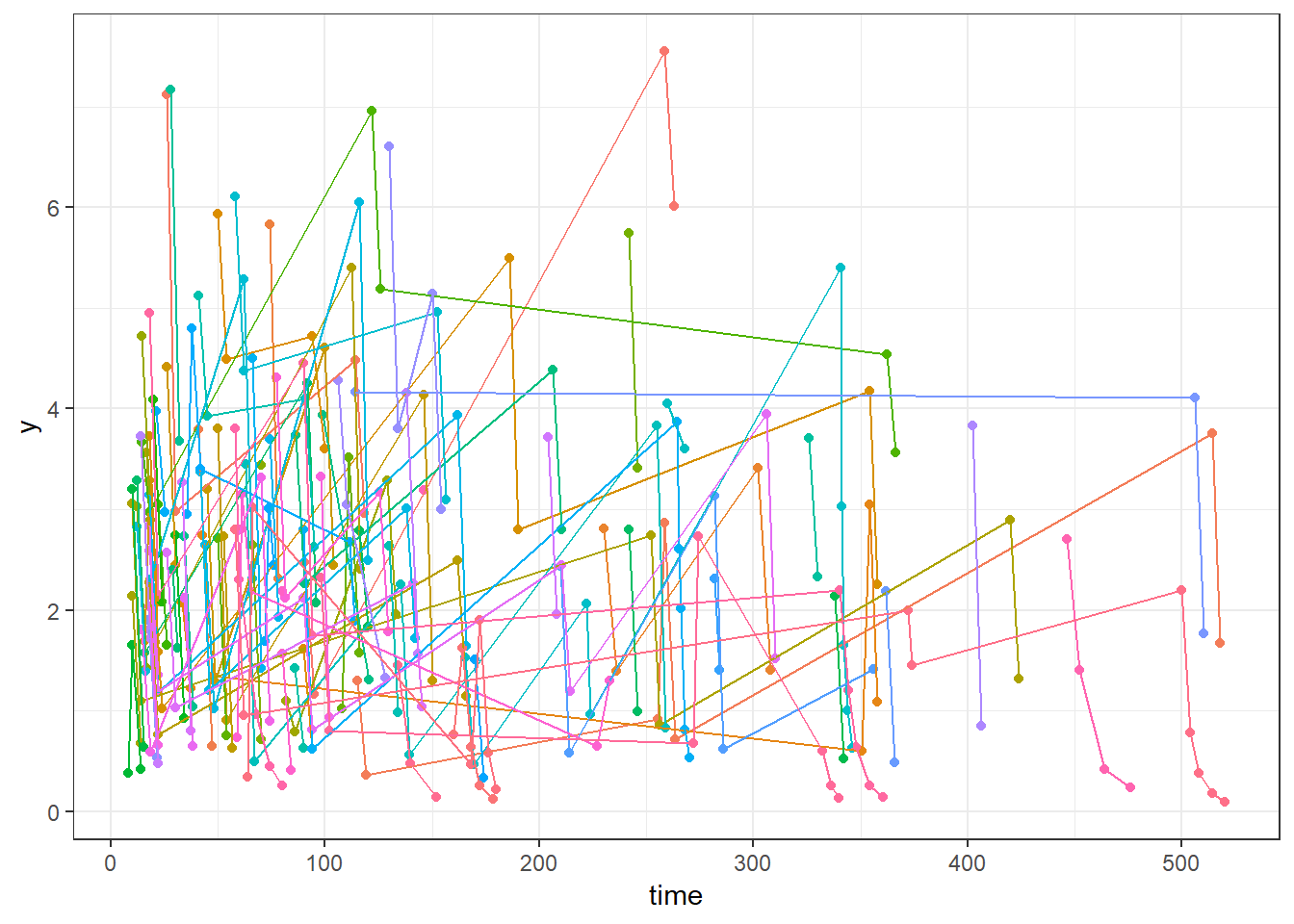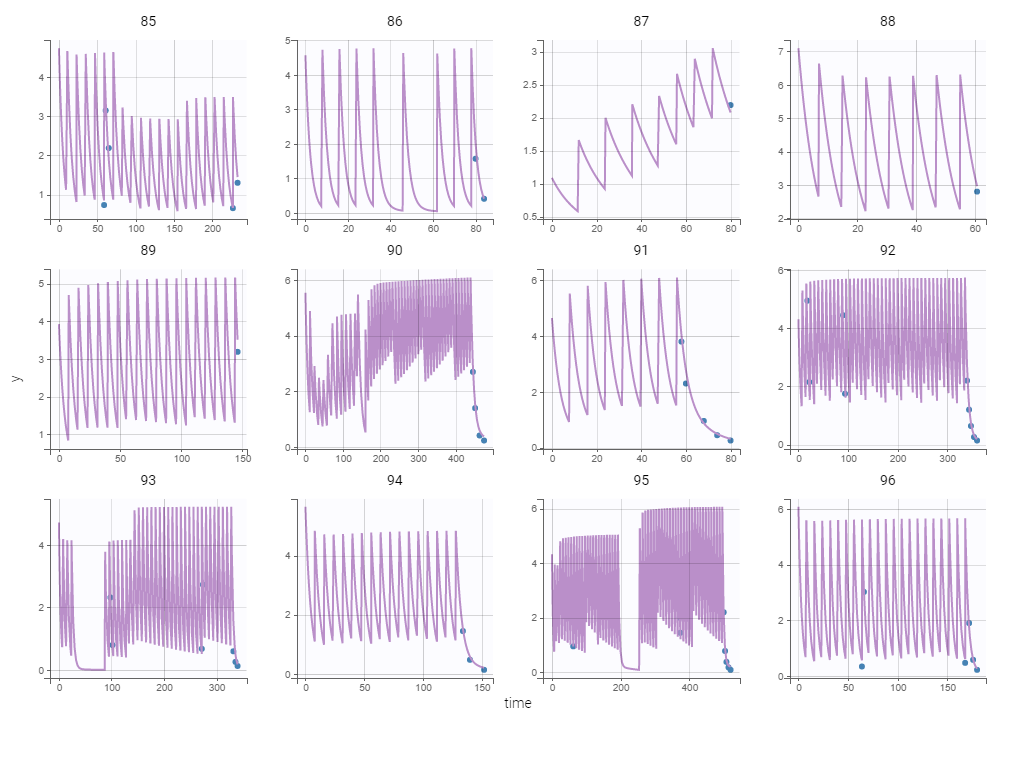library(Rsmlx)
library(ggplot2)
theme_set(theme_bw())

Let us first read and plot the tobramycin data

tobramycin <- list(
dataFile = "data/tobramycin.csv",
headerTypes = c("id", "time", "observation", "amount", "evid", "contcov", "contcov", "contcov", "catcov"),

ggplot(tobramycin.data$y, aes(x=time, y=y, color=id)) + geom_point() + geom_line() + theme(legend.position = "none")Get some good initial estimates pkpopini gives some very good initial estimates for a 2 compartment model: r2 <- pkpopini(parameter=c("k", "V", "k12","k21"), data=tobramycin, new.dir="tobramycin") print(r2$pop.ini)
##           k           V         k12         k21
##  0.21657057 18.93801764  0.04220483  0.07414199

The Monolix project “tobramycin/pk_Vk12k21k.mlxtran” was also created by pkpopini. This project could now be loaded and run in Monolix.

Despite very sparse data, initial values for a 3 compartment model can also be obtained with pkpopini:

r3 <- pkpopini(parameter=c("k", "V", "k12","k21", "k13","k31"), data=tobramycin, new.dir="tobramycin")
print(r3$pop.ini) ## k V k12 k21 k13 k31 ## 0.21658919 18.93817780 0.01995253 0.07414982 0.02232342 0.07419577 Select the structural model We use pkbuild to build a PK model for this data, using transfer rates as parameterization: tobramycin.rate <- pkbuild(data=tobramycin, param="rate", new.dir="tobramycin") ## ##  "tobramycin/pk_Vk.mlxtran" ## Estimation of the population parameters... ## Estimation of the log-likelihood... ## ##  "tobramycin/pk_Vk12k21k.mlxtran" ## Estimation of the population parameters... ## Estimation of the log-likelihood... ## ##  "tobramycin/pk_Vk12k21k13k31k.mlxtran" ## Estimation of the population parameters... ## Estimation of the log-likelihood... According to (corrected) BIC, the best PK models for this data are the following ones print(tobramycin.rate$bic)
##                              model  bic.cor
## 1       infusion_2cpt_Vkk12k21.txt 693.0616
## 2             infusion_1cpt_Vk.txt 699.5475
## 3 infusion_3cpt_Vkk12k21k13k31.txt 717.5586

Using Monolix to fit the data with the selected model

The following Monolix project was created by pkbuild

tobramycin.rate\$project
##  "tobramycin/pk_Vk12k21k.mlxtran"

We can load and run this project with Monolix (either using the lixoftConnectors or the Monolix graphical user interface). Here are some individual fits obtained with Monolix: